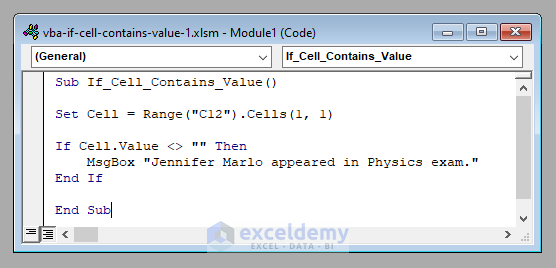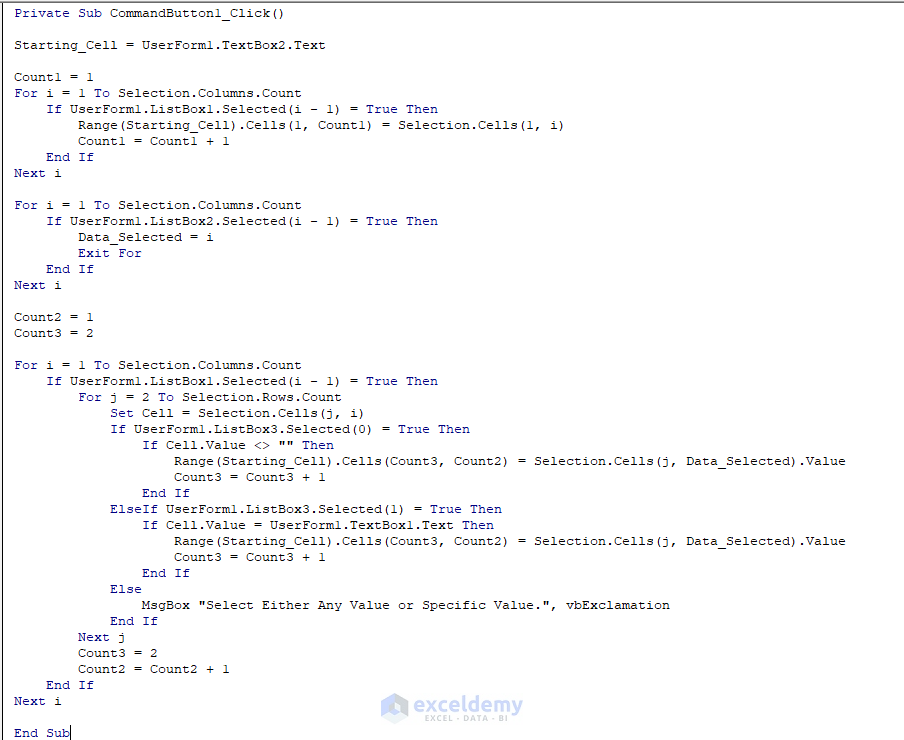# Excel VBA: If Cell Contains Value Then Return a Specified Output

Get FREE Advanced Excel Exercises with Solutions!

In this article, I’ll show you how you can analyze the situation in Excel VBA if a cell contains a value. You’ll learn to analyze the situation for both any value and a particular value.

Excel VBA Analysis: If Cell Contains a Value then (Quick View)

``````Sub If_Cell_Contains_Value()

Set Cell = Range("C12").Cells(1, 1)

If Cell.Value <> "" Then
MsgBox "Jennifer Marlo appeared in Physics exam."
End If

End Sub``````

## Excel VBA Analysis: If Cell Contains a Value Then Return Outputs (Step by Step Analysis)

Here we’ve got a data set with the marks of some students in Physics, Chemistry, and Mathematics in a school called Sunflower Kindergarten.The blank cells mean that the student didn’t appear, that is, was absent in that particular exam. Now let’s analyze the situation, whether a cell contains a value or not, with this data set.

⧪ Step 1: Declaring the Cell

First, we have to declare the cell whose value we want to analyze. Let’s analyze whether Jennifer Marlo appeared in the Physics exam or not.

Therefore, we need to check whether cell C12 contains a value or not.

To do that, first, we have to declare cell C12.

The line of code to accomplish this will be:

``Set Cell = Range("C12").Cells(1, 1)``

⧪ Step 2: Checking If the Cell Contains a Value (Including a Particular Value)

Next, we’ve to check whether the cell contains a value or not. We’ll execute this using an If condition. The line of  code will be:

``If Cell.Value <> "" Then``

This code will be executed if the cell contains any value. To check for a value (For example, whether it contains 100 or not), use that specific value with an Equal to symbol.

``If Cell.Value <> "" Then``

⧪ Step 3: Allotting the Task

Then you’ve to set up a task that’ll be executed if the cell contains a value (or a specific value).

Here, we want to display the message “Jennifer Marlo appeared in Physics exam.”. So the line of code will be:

``MsgBox "Jennifer Marlo appeared in Physics exam."``

⧪ Step 4: Ending the If Block

Finally, you have to declare an end to the If block.

``End If``

So the complete VBA code will be:

⧭ VBA Code:

``````Sub If_Cell_Contains_Value()

Set Cell = Range("C12").Cells(1, 1)

If Cell.Value <> "" Then
MsgBox "Jennifer Marlo appeared in Physics exam."
End If

End Sub``````⧭ Output:

Run this code by clicking the Run Sub/UserForm button on the toolbar.It’ll display the message “Jennifer Marlo appeared in Physics exam.” as the cell C12 contains a value, of 50.## Examples Involving If Cell Contains a Value Then a Specified Output with Excel VBA

We’ve learned to analyze if a cell contains a value or not in VBA. Now, let’s explore some examples to make the understanding clear.

### 1. Developing a Macro to Filter Out a Value If the Corresponding Cell Contains any Value then in Excel VBA

Let’s develop a Macro to filter out the students who appeared in each of the exams.

That is, we have to check the cells containing the marks of each of the subjects and see whether they contain a value or not.

If they do, then we have to sort out the name of the corresponding student.

The complete VBA code to accomplish this will be:

⧭ VBA Code:

``````Sub Sorting_Out_Cells_that_Contain_Values()

Starting_Cell = InputBox("Enter the Reference of the First Cell of the Filtered Data: ")

For i = 2 To Selection.Columns.Count
Range(Starting_Cell).Cells(1, i - 1) = Selection.Cells(1, i)
Next i

Count = 2

For i = 2 To Selection.Columns.Count
For j = 2 To Selection.Rows.Count
Set Cell = Selection.Cells(j, i)
If Cell.Value <> "" Then
Range(Starting_Cell).Cells(Count, i - 1) = Selection.Cells(j, 1).Value
Count = Count + 1
End If
Next j
Count = 2
Next i

End Sub``````⧭ Output:

Select the data set (Including the Headers) and run this Macro.First, you’ll be asked to enter the reference of the first cell where you want the filtered data. I’ve entered G3.Then click OK. You’ll get the names of the students who appeared in each of the exams (Including the Headers) in a new data set starting from cell G3.### 2. Creating a User-Defined Function to Sort Out a Value If the Corresponding Cell Contains a Specific Value then in Excel VBA

Now we’ll create a user-defined function that will return the names of the students who got a specific mark in each of the subjects.

The procedure is almost the same as the above. We have to check the cells containing the marks of each of the subjects and see whether they are equal to a specific value or not.

If they are, then we’ll return the name of the corresponding student.

The VBA code will be:

⧭ VBA Code:

``````Function Cells_with_Values(Rng As Range, Data As Variant)

Dim Output() As Variant
ReDim Output(Rng.Rows.Count, Rng.Columns.Count - 1)

For i = 0 To Rng.Columns.Count - 2
Output(0, i) = Rng.Cells(1, i + 2)
Next i

Count = 1

For i = 2 To Rng.Columns.Count
For j = 2 To Rng.Rows.Count
Set Cell = Rng.Cells(j, i)
If Cell.Value = Data Then
Output(Count, i - 2) = Rng.Cells(j, 1).Value
Count = Count + 1
End If
Next j
Count = 1
Next i

For i = LBound(Output, 1) To UBound(Output, 1)
For j = LBound(Output, 2) To UBound(Output, 2)
If Output(i, j) = 0 Then
Output(i, j) = ""
End If
Next j
Next i

Cells_with_Values = Output

End Function``````⧭ Output:

The code creates a function called Cells_with_Values that takes two arguments, a Range and a Value.

Let’s find out the students who got 100 in each of the subjects using this function.

Select a range of cells in your worksheet and enter this function in the first cell of the range:

`=Cells_with_Values(B3:E13,100)`
[Here B3:E3 is my data set (Including the Headers) and 100 is my matching value. You use your one.]Then press CTRL + SHIFT + ENTER (Array Formula). It’ll return an array consisting of the names of the students who got 100 in each subject, including the Headers.### 3. Developing a UserForm to Extract Out a Value if the Corresponding Cell Contains any Value (Or a Specific Value) in Excel VBA

Finally, we’ll develop a UserForm to extract the names of students who appeared (or got specific marks) in some specific exams.

⧪ Step 1: Opening the UserForm

Go to the Insert > UserForm option in the VBA editor to open a new UserForm. A new UserForm called UserForm1 will be opened.⧪ Step 2: Dragging Tools to the UserForm

Besides the UserForm, you’ll get the Toolbox. Move your cursor over the Toolbox and drag 4 Labels (Label1, Label2, Label3, Label4) and 3 ListBoxes (ListBox1, ListBox2, ListBox3) and a TextBox (TextBox1) in a rectangular shape over the UserForm.

Then drag another Label (Label5) and a TextBox (TextBox2) to the bottom left corner of the UserForm.

Finally, drag a CommandButton (CommandButton1) to the bottom right corner.

Change the displays of the Labels to Lookup Column, Return Column, Any Value or a Specific Value, Value, and Starting Cell.

Also, change the display of the CommandButton1 to OK.⧪ Step 3: Writing Code for ListBox3

Double click on ListBox3. A Private Subprocedure called ListBox3_Click will open. Enter the following code there.

``````Private Sub ListBox3_Click()

If UserForm1.ListBox3.Selected(0) = True Then
UserForm1.Label4.Visible = False
UserForm1.TextBox1.Visible = False

ElseIf UserForm1.ListBox3.Selected(1) = True Then
UserForm1.Label4.Visible = True
UserForm1.TextBox1.Visible = True
End If

End Sub``````⧪ Step 4: Writing Code for CommandButton1

Then double click on CommandButton1. Another Private Subprocedure called CommandButton1_Click will open. Enter the following code there.

``````Private Sub CommandButton1_Click()

On Error GoTo Message

Starting_Cell = UserForm1.TextBox2.Text

Count1 = 1

For i = 1 To Selection.Columns.Count

If UserForm1.ListBox1.Selected(i - 1) = True Then

Range(Starting_Cell).Cells(1, Count1) = Selection.Cells(1, i)

Count1 = Count1 + 1

End If

Next i

If Count1 = 1 Then
MsgBox "Select at Least One Lookup Column.", vbExclamation
Exit Sub
End If

Data_Selected = 0

For i = 1 To Selection.Columns.Count
If UserForm1.ListBox2.Selected(i - 1) = True Then
Data_Selected = i
Exit For
End If
Next i

If Data_Selected = 0 Then
MsgBox "Select One Return Column.", vbExclamation
Exit Sub
End If

Count2 = 1

Count3 = 2

For i = 1 To Selection.Columns.Count
If UserForm1.ListBox1.Selected(i - 1) = True Then
For j = 2 To Selection.Rows.Count
Set Cell = Selection.Cells(j, i)
If UserForm1.ListBox3.Selected(0) = True Then
If Cell.Value <> "" Then
Range(Starting_Cell).Cells(Count3, Count2) = Selection.Cells(j, Data_Selected).Value
Count3 = Count3 + 1
End If
ElseIf UserForm1.ListBox3.Selected(1) = True Then
If Cell.Value = UserForm1.TextBox1.Text Then
Range(Starting_Cell).Cells(Count3, Count2) = Selection.Cells(j, Data_Selected).Value
Count3 = Count3 + 1
End If
Else
MsgBox "Select Either Any Value or Specific Value.", vbExclamation
Exit For
End If
Next j
Count3 = 2
Count2 = Count2 + 1
End If
Next i

Exit Sub

Message:
MsgBox "Enter a Valid Cell Reference as the Starting Cell.", vbExclamation

End Sub``````

⧪ Step 5: Writing Code for Running the UserForm

Finally, insert a new Module from the VBA toolbar and insert the following code there.

``````Sub Run_UserForm()

UserForm1.Caption = "Filtering Cells that Contain Values"

UserForm1.ListBox1.BorderStyle = fmBorderStyleSingle
UserForm1.ListBox1.ListStyle = fmListStyleOption

UserForm1.ListBox2.BorderStyle = fmBorderStyleSingle
UserForm1.ListBox2.ListStyle = fmListStyleOption

UserForm1.ListBox3.BorderStyle = fmBorderStyleSingle
UserForm1.ListBox3.ListStyle = fmListStyleOption

For i = 1 To Selection.Columns.Count
Next i

UserForm1.ListBox1.MultiSelect = fmMultiSelectMulti

UserForm1.Label4.Visible = False

UserForm1.TextBox1.Visible = False

UserForm1.Show

End Sub``````⧪ Step 6: Running the UserForm (The Final Output)

Your UserForm is now ready to use. Select the data set from the worksheet (B3:E13 here) (Including the Headers) and run the Macro called Run_UserForm.The UserForm will be loaded in the worksheet. From the Lookup Column table, I’ve selected Physics and Mathematics, because I want to get the student names who appeared in Physics and Mathematics exams.

From the Return Column table, I’ve selected Student Name, because I want to get the student names.

And from the Any Value or Specific Value table, I’ve selected Any Value.

Finally, in the Starting Cell box, I have put G3.Then click OK. You’ll get the names of the students who appeared in Physics and Mathematics exams starting from cell G3.If you had selected Specific Value from the Any Value or Specific Value table, you would have got another TextBox to enter the specific value.

Here I’ve entered 100.Click OK. And you’ll get the students who got 100 in Physics and Chemistry.## Things to Remember

We used a two-dimensional array to return the student names in the User-Defined Function described in example 2.

## Conclusion

So these are a lot of things regarding analyzing if a cell contains a value or not, isn’t it? Hope the examples will make things clear for you. Still, if you have any questions, feel free to ask us. And don’t forget to visit our site ExcelDemy for more posts and updates.

## Related Articles#### Rifat Hassan

Hello! Welcome to my profile. Here I will be posting articles related to Microsoft Excel. I am a passionate Electrical Engineer holding a Bachelor’s degree in Electrical and Electronic Engineering from the Bangladesh University of Engineering and Technology. Besides academic studies, I always love to keep pace with the revolution in technology that the world is rushing towards day by day. I am diligent, career-oriented, and ready to cherish knowledge throughout my life.

We will be happy to hear your thoughtsAdvanced Excel Exercises with Solutions PDF